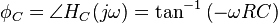# Voltage relations in a circuit

FutileSentiet
Hi there,

I am writing up a labratory report at the moment and I am a little confused about the phase realtive to v(capacitor) relative to v(resistor). In a circuit with a capacitor and a resistor, how will the phase change when the frequency is changed?

Say from 100Hz to 1kHz to 10kHz, how will the phase of v(capacitor) change realtive to v(resistor)? Assuming the resistor is 15.91kOhms and the capacitor is 0.010microF.

Thanks, I have been trying to find some infromation on this but have been unsucessful.

Homework Helper
I suppose the capacitor and resistor are connected in series to the ac generator. In that case, the current is the same on both. What do you know about the phase between current and voltage across a resistor and phase between current and voltage across a capacitor?

ehild

Homework Helper
Gold Member
FutileSentiet
I suppose the capacitor and resistor are connected in series to the ac generator. In that case, the current is the same on both. What do you know about the phase between current and voltage across a resistor and phase between current and voltage across a capacitor?

ehild

The current and voltage are out of phase by 90 degrees on a circuit with just a capacity. And that the voltage and current are in phase on a ciruit with just a resistor, I do believe. I am still not sure how these two react when placed in the same circuit.

FutileSentiet
Perhaps see..
http://en.wikipedia.org/wiki/RC_circuit

Has equations for the phase.

From what I see there it only gives the phase relation to voltage in, unless perhaps you coukd piont it out to me.

Homework Helper
Gold Member
See "The magnitude of the gains across the two components are:" and "and the phase angles are"FutileSentiet
See "The magnitude of the gains across the two components are:" and "and the phase angles are"Those phase angles are relative to the voltage in though aren't they?

Last edited by a moderator:
Homework Helper
Gold Member
So subtract one from the other?

FutileSentiet
So subtract one from the other?

I'm pretty sure you'd actually have to add them since they are relative to voltage in, this would get the total angle between them.

Would this mean that the phase between them is 90 degrees? And I guess this would also mean that the voltage in wouldn't be 90deg out of phase as it would in a circuit with just a capacitor.

I also need to know how this relationship will change with a higher frequency.

Homework Helper
The current and voltage are out of phase by 90 degrees on a circuit with just a capacity. And that the voltage and current are in phase on a ciruit with just a resistor, I do believe. I am still not sure how these two react when placed in the same circuit.

If the same current flows through the capacitor and through the resistor, and the voltage across the resistor is in phase with the current and the voltage across the capacitor is 90° behind the same current, what is the phase of Vc with respect to VR?

You can also speak about the phase difference between the generator voltage and current, but you did not ask that.

ehild

FutileSentiet
If the same current flows through the capacitor and through the resistor, and the voltage across the resistor is in phase with the current and the voltage across the capacitor is 90° behind the same current, what is the phase of Vc with respect to VR?

You can also speak about the phase difference between the generator voltage and current, but you did not ask that.

ehild

Ok so it is out of phase by 90°, but how does the change when the frequency is changed? From a low frequency to one that is above the 3-d B point?

Homework Helper
Constant phase difference does not change with frequency.

ehild